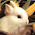Sunday, October 3, 2010

Count the number of ways .

You have n number of chocolates. Your best friend asks for the chocolate but you say that he can take only 1 or maximum 2 chocolates at a time.
Find the number of ways in which your friend can take all the chocolates in any number of times.

Approach :

The first thing to note is that how can you take n chocolates ?
Before having n chocolates you need to have either n-1 or n-2 chocolates (as you can only pick 1 or 2 chocolates).

So if f(n) denotes the number of ways to pick n chocolates then
f(n) = f(n-1) + f(n-2)
Wow it turns out to be a Fibonacci series !!!
Base cases you can work it out  :)

Try the extension of this problem here

1.int no_of_ways(int total_coins)
{
return (no_of_ways(total_coins-1) + no_of_ways(total_coins-2) );
}

2.In simple terms.... it is a Fibonacci series :)

3.sir,
ek dum hit nahi howz it fibonacci
shashwat sinha

4.It can be understood if u look at the problem as a DP. Since u can give only 1 or 2 chocolates at a time so the number of ways can be computed based on the earlier computations. If
Number of ways of giving n chocolates = f(n)
So f(n) = f(n-1) + f(n-2) which is a Fibonacci series.

Regards